# Excel Applications for Accounting Principles

## Quiz 24 : Material and Labor Variances PrimevarLooking for Accounting Homework Help?

## Quiz 24 :Material and Labor Variances Primevar

Question TypePROBLEM DATA McGrade Industries manufactures a product whose standard direct materials and direct labor unit costs are as follows: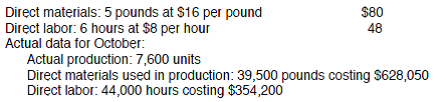REQUIREMENT What is the total actual cost of October's production Based on the standards, what should it have cost Compute the total difference (variance) between these two amounts.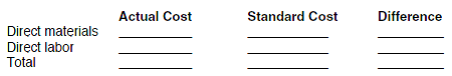Free
Essay

Review the worksheet indicated in the problem. Review the problem data. Based on the data, calculate the actual cost, standard cost, and the difference for direct materials and direct labor. To do this, you will need to multiply standard rates for materials and labor by the actual number of units produced.
Once you have made all the calculations, you should have this information: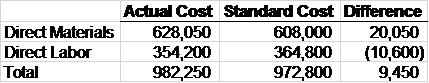TagsPROBLEM DATA McGrade Industries manufactures a product whose standard direct materials and direct labor unit costs are as follows:REQUIREMENT The president of McGrade Industries wants an analysis prepared to help explain why the variances computed in requirement 1 occurred. Using the worksheet called PRIMEVAR that follows these requirements, calculate the material and labor variances for McGrade Industries. The problem requires you to enter the input in the Data Section as well as formulas in the Answer Section.
Free
Essay

Review the worksheet indicated. Input your data where indicated in the worksheet using your calculations from the given data. When you finish the data section, your worksheet should look like this: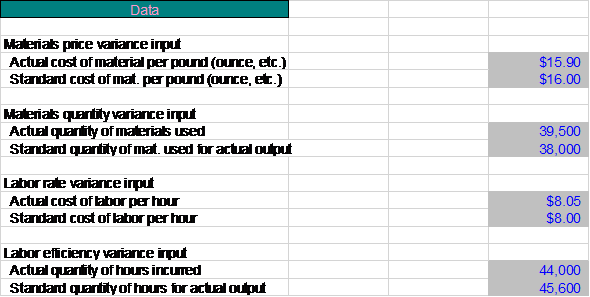TagsPROBLEM DATA McGrade Industries manufactures a product whose standard direct materials and direct labor unit costs are as follows:REQUIREMENT Open the file PRIMEVAR from the website for this book at cengagebrain.com. Enter the input values or formulas to compute the input values. Then enter the formulas where indicated on the worksheet. As a hint, FORMULA1 can be written as =(D8-D9)*D12. Formulas 8-10 should enter an F if the variance is favorable and a U if it is unfavorable. See the discussion of the =IF function in Appendix A of Excel Quick. Enter your name in cell A1. Save the completed file as PRIMEVAR3. Print the worksheet when done. Also print your formulas. Check figure: Material quantity variance (cell B27), \$24,000 U.
Free
Essay

Open the file indicated in the instructions. Input the information from the worksheet into the data section of the file. In the answers section of the file, enter the formulas to calculate the materials and labor variance for this product using cell references so that the file will automatically update if any data is changed. Use the IF formula to indicate an "F" for a favorable variance and an "U" for unfavorable variance. A positive number is indicative as an unfavorable variance while a negative number indicates a favorable variance.
When you have finished both the data and answers section, your file should look like this: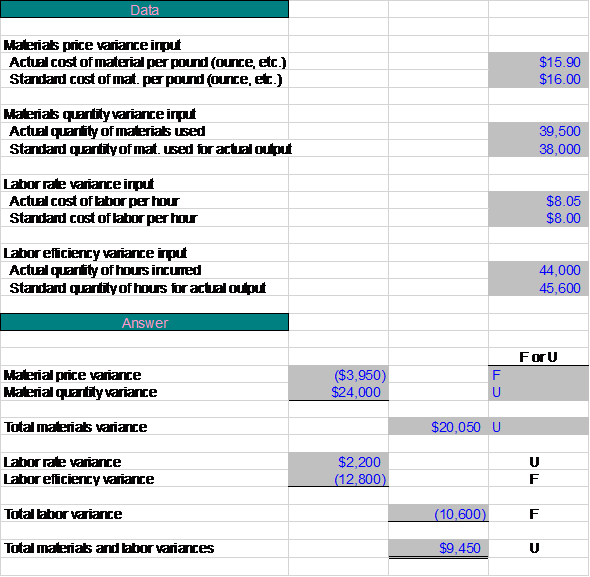TagsWHAT-IF ANALYSIS The worksheet you have developed will handle most simple variance analysis problems. Try the problem below for Pscheidl, Inc.: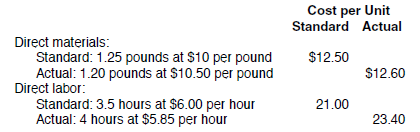Actual production for October was 11,500 units. Compute the direct materials and direct labor variances for Pscheidl, Inc. Be careful when entering your input because this problem presents the information in a different format from the McGrade Industries' data. Save the file as PRIMEVAR4. Print the worksheet when done.
Essay